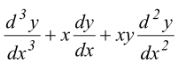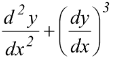# Introduction

## Differential Equations of Class 12

### Definition

Equation involving independent variable, dependent variable and derivatives of dependent variable with respect to independent variable is called differential equation.

### Order of a differential equation

The order of highest order derivative appearing in a differential equation is said to be order of differential equation.

For example differential equation+ 4 = 0 is of order 3. And differential equation= 0 is of order 2.